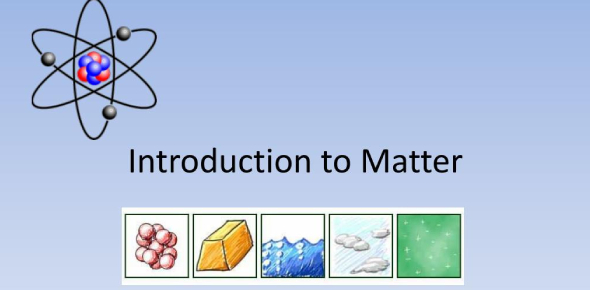# Introduction To Matter Practice Questions! Quiz

22 Questions | Total Attempts: 1277Settings.

• 1.
Which type of matter consists of two or more substances that are NOT chemically combined?
• A.

Atoms

• B.

Mixtures

• C.

Elements

• D.

Compounds

• 2.
Substances that CANNOT be broken down into other substances are called __________.
• A.

Compounds

• B.

Liquids

• C.

Mixtures

• D.

Elements

• 3.
In chemistry, elements are represented by _________________.
• A.

Building blocks

• B.

Formulas

• C.

Symbols

• D.

Ratios

• 4.
The measurement of how much matter an object contains is its _________________.
• A.

Density

• B.

Weight

• C.

Mass

• D.

Volume

• 5.
Which of the following is NOT true of a compound?
• A.

It contains elements combined in a specific ratio.

• B.

It is made up of two or more elements.

• C.

It is made of substances that keep their individual properties.

• D.

It contains elements that are chemically combined.

• 6.
The SI unit for mass is the ___________________.
• A.

Kilogram

• B.

Ounce

• C.

Liter

• D.

Pound

• 7.
How would you calculate the density of an object?
• A.

Multiply its weight times its mass

• B.

Divide its weight by its volume

• C.

Divide its mass by its volume

• D.

Multiply its volume times its mass

• 8.
What is the density of a block of wood with a volume of 10 cubic centimeter and a mass of 100 grams?
• A.

10 grams/cubic centimeter

• B.

100 grams/cubic centimeter

• C.

1 gram/cubic centimeter

• D.

10 cubic centimeters/gram

• 9.
All elements are composed of extremely small particles called _______________.
• A.

Molecules

• B.

Atoms

• C.

Mixtures

• D.

Compounds

• 10.
A group of atoms that are bonded together are called __________________.
• A.

Mixtures

• B.

Compounds

• C.

Elements

• D.

Molecule

• 11.
What holds atoms together in a molecule?
• A.

Temperature

• B.

Chemical bonds

• C.

Gravity

• D.

Density

• 12.
What technique did miners use to separate dirt from gold?
• A.

Heating along with charcoal

• B.

Electrolysis

• C.

Melting

• D.

Panning

• 13.
What does this diagram represent?
• A.

Molecules

• B.

An atom

• C.

An element

• D.

Nothing

• 14.
What does this picture represent?
• A.

A homogeneous mixture

• B.

An element

• C.

A heterogeneous mixture

• D.

A pure substance

• 15.
What does this picture represent?
• A.

A pure substance

• B.

A mixture

• C.

A compound

• D.

None of the above

• 16.
Which of the following examples below represents a homogeneous mixture?
• A.

• B.

A trash dump

• C.

• D.

A glass of water

• 17.
What shows the ratio of elements in a compound?
• A.

A mixture

• B.

Element symbols

• C.

A formula

• D.

A compound

• 18.
Most matter occurs in the environment as _________________.
• A.

Elements

• B.

Compounds

• C.

Mixtures

• D.

Atoms

• 19.
The measure of the force of gravity on an object is called its _______________.
• A.

Mass

• B.

Length

• C.

Weight

• D.

Height

• 20.
True or False -- The density of a substance varies with the samples of that substance.
• A.

True

• B.

False

• 21.
The measurement of the amount of space an object takes up is its ______________.
• A.

Volume

• B.

Density

• C.

Formula

• D.

Mass

• 22.
A change in matter that produces new substances is called a _________________.
• A.

Physical change

• B.

Mental change

• C.

Chemical change

Related TopicsBack to top